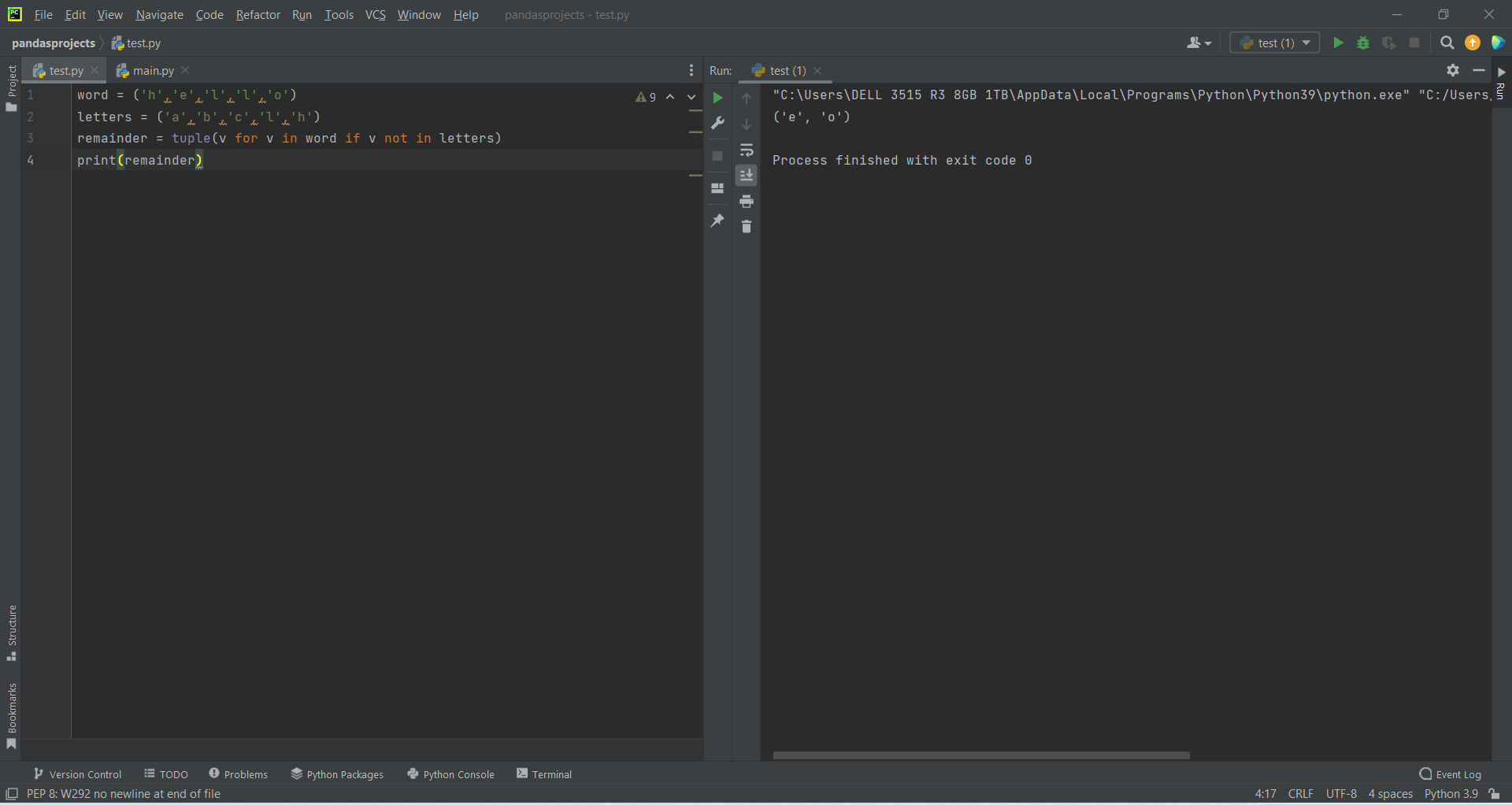# How to Create a Set Functions with Tuples in Pythonby Abdul Rawoof A RUpdated: Jan 31, 2023Guide Kit

Commas separate the elements of a tuple. A tuple is a collection of ordered, immutable elements in Python It is like a list but enclosed in parentheses instead of square brackets.

A set function is a function that assigns a value or set of values to each element of a given set. For example, the function that assigns a value of "even" to each even number and "odd" to each odd number in the set of natural numbers is a set function.

In Python, set functions can be used with tuples in several ways. Here are a few examples:

• The built-in “len()” function can find the number of elements in a tuple.
• The “max()” and “min()” functions can find the maximum and minimum elements in a tuple, respectively.
• The “sum()” function can find the sum of all elements in a tuple of numbers.
• The “count()” method can calculate the number of occurrences of a specific element in a tuple.
• The “in” keyword can check if an element is present in a tuple.Fig : Preview of the output that you will get on running this code from your IDE.

### Instructions

Follow the steps carefully to get the output easily.

2. Copy the snippet using the 'copy' and paste it in your IDE.
3. Run the file to generate the output.

I hope you found this useful.

I found this code snippet by searching for 'set function with tuples in python' in kandi. You can try any such use case!

### Environment Tested

I tested this solution in the following versions. Be mindful of changes when working with other versions.

1. The solution is created in PyCharm 2021.3.
2. The solution is tested on Python 3.9.7.

Using this solution, we are able to implement set functions with tuples in python with simple steps. This process also facilities an easy way to use, hassle-free method to create a hands-on working version of code which would help us to implement set functions with tuples in python.

### Support

1. For any support on kandi solution kits, please use the chat
2. For further learning resources, visit the Open Weaver Community learning page.